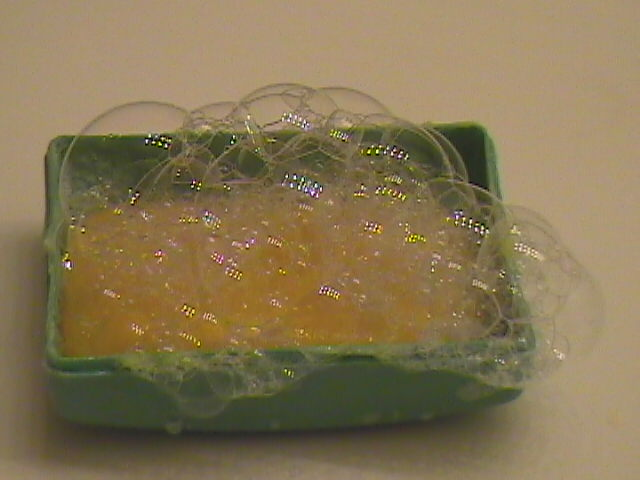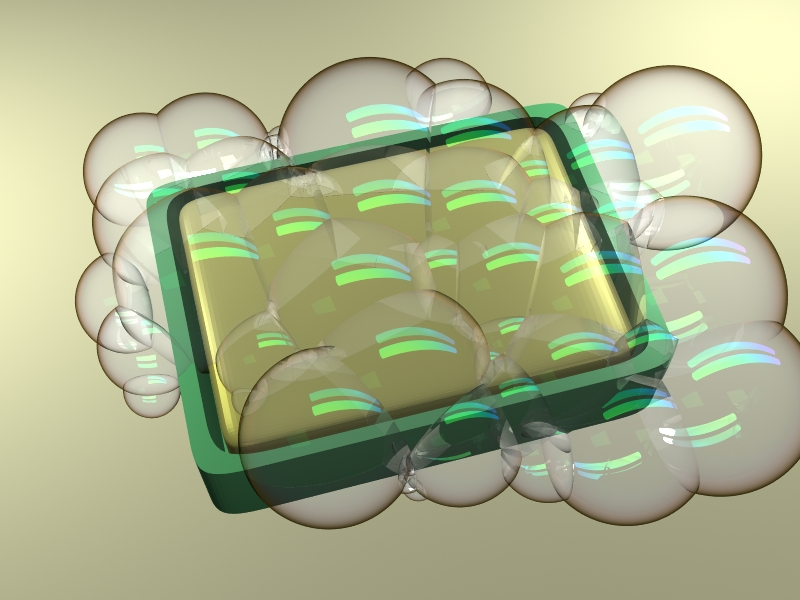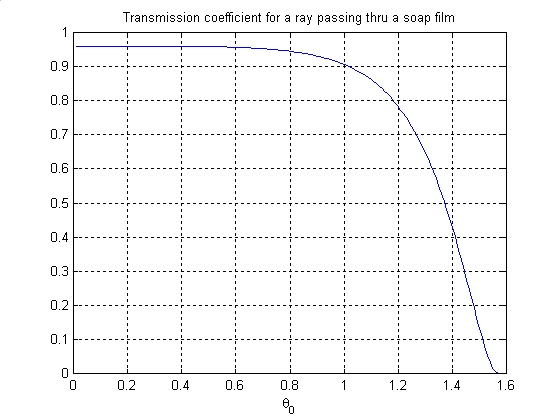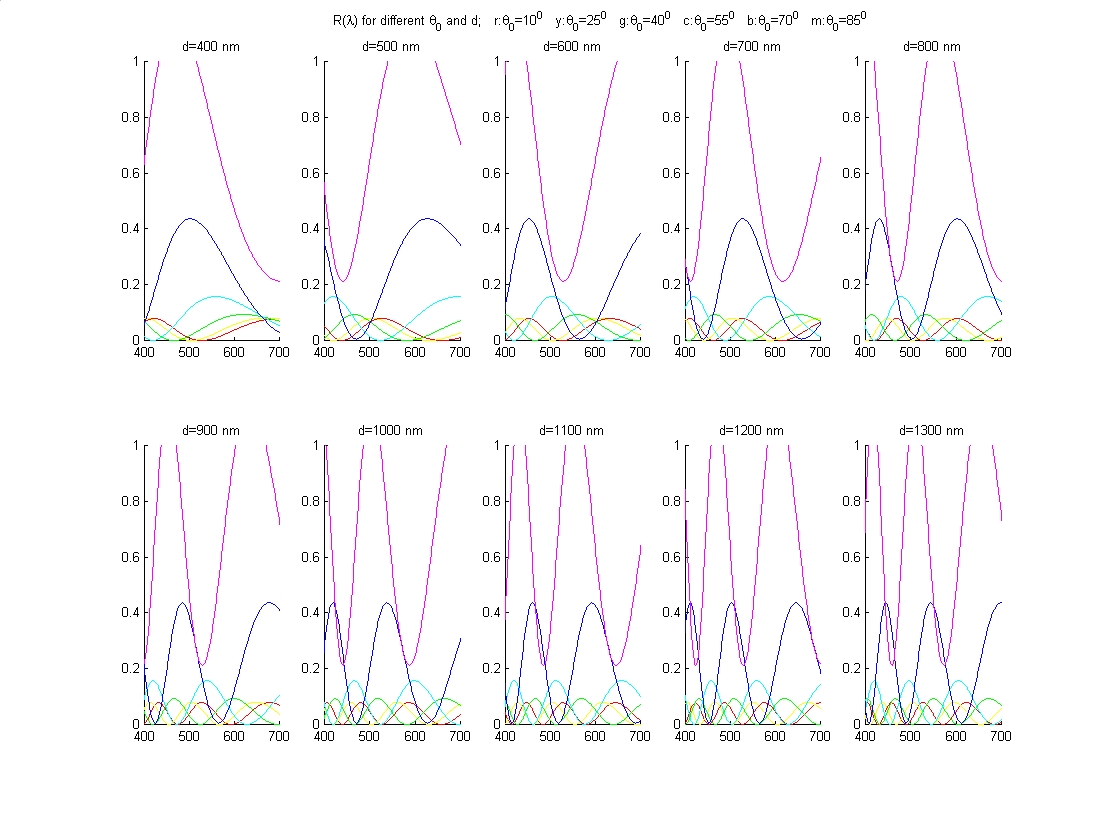How to make soap using simple household items

The goal of my project was to render soap bubbles. This required to model the optical properties of soap film and the geometric arrangement of a cluster of bubbles, and to implement a data structure for ray-bubble cluster intersection.

Original picture:                                                                                                             Rendering:Some more pictures, modeling soap with varying degree of success:Optical properties
I considered scattering of a ray of light of wavelength lambda (ranges from 380 to 780 nm) by a soap film of thickness d (useful range ~ 500 - 1000 nm) and with index of refraction eta = 1.33; angle of incidence theta0 ranges from 0 to 90 degrees. I looked up the formulas for the coefficients of transmission and reflection: R_{perp, parallel}(theta0,eta), T_{perp,parallel}(theta0,eta), where "parallel" pertains to the light polarized in the plane of incidence, and "perp" - polarized perpendicular to the plane of incidence.
I largely neglected the polarization effects and lumped together the two kinds of rays, except that I separately computed the reflection with interference for the two types of polarization and only then added them. I also neglected the dependence of the index of refraction on the angle of incidence (which is not strong), and I only considered the rays that go through the film back and forth once (transmission - internal reflection - transmission). Thus I only had two fainter (and coherent) versions of an incident ray to engage in interference with each other.

Here plot T(theta0), R(lambda) for d = 500:100:1000, for a couple of theta0Naturally I had to use a more detailed representation of a light ray than just RGB. I found a table of CIE  XYZ functions on the web at www.cvrl.org, and also found a matrix for conversion of XYZ coordinates to RGB. (not necessarily those corresponding to my monitor, but they were good enough)  In lrt I created an extra spectrum class which carried a spectral distribution discretized for lambda in the range 380-780 nm with step 5nm. This class was responsible for converting the output of my reflectance function, which strongly depends on lambda, to RGB.

Geometric properties
Given two joined bubbles of radii R and r, we can immediately infer the radius of the wall between them from the law for the pressure increase due to surface tension in liquid. It is 1/r_between = 1/r - 1/R.
Thus we can seed some spheres in space and compute physically realistic parameters for the partitions. But not every sphere arrangement corresponds to a physically stable configuration of a bubble cluster.
Bubbles strive to form the angle of 120 degrees with each other, which translates to the desirable distance d=sqrt(R*R - R*r + r*r). We could neglect this circumstance, but then the cluster will lose part of its realism, the kind of  look you find in a Voronoi diagram. I suppose the inside of a pomegranate is another example of the same arrangement.

To incorporate this phenomenon into my model, I implemented a relaxation process for the bubble cluster, which I monitored interactively in OpenGL. Conceptually we assume that we somehow are given the final bubble radii (which of course change in reality as the bubbles group) and an approximate arrangement and try to solve for the exact arrangement. Essentially I told each bubble to find the desired displacements that each neighbor alone would ask this bubble to make, then average them and move accordingly.  With this rule alone the bubbles tended to draw together too much, so I had to tweak the relaxation procedure a bit.

Here are some pics from my OpenGL demo

Intersection routines
To intersect a ray with a bubble cluster, I first detect whether the ray originates outside of cluster; if yes, I just intersect the ray with the bubble spheres independently.
If a ray originates inside a bubble cluster, I find the bubble containing the ray origin (I use the exact physically dictated radius of wall, and fit this spherical cap / disk to the intersection of the bubble spheres, which may not be positioned so as to form the angle 120 degrees with each other), and then intersect the ray with the walls and the original sphere of this bubble independently. Since the walls are always of larger radius then the original sphere of the bubble, I don't have to explicitly store the boundaries of the walls, just the spheres (and planes in case of neighbors of equal radii), although for the OpenGL interaction I explicitly computed the sphere caps and disks.